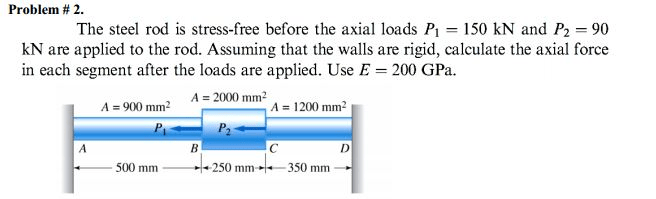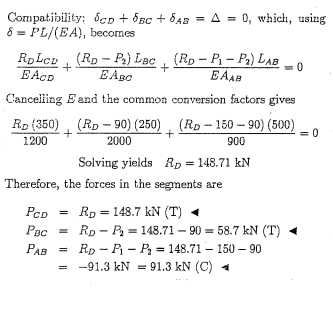# The steel rod is stress-free before the axial loads P1 = 150 kN and P2 =90 kN am applied to the rod. Assuming that the walls am rigid, calculate the axial force in each segment after the loads are applied. Use E = 200 GPa

Question-AnswerCategory: Strength of MaterialsThe steel rod is stress-free before the axial loads P1 = 150 kN and P2 =90 kN am applied to the rod. Assuming that the walls am rigid, calculate the axial force in each segment after the loads are applied. Use E = 200 GPaProblem #2. The steel rod is stress-free before the axial loads P1 = 150 kN and P2 =90 kN am applied to the rod. Assuming that the walls am rigid, calculate the axial force in each segment after the loads are applied. Use E = 200 GPa